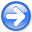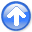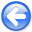Tomographic full waveform inversion and linear modeling of multiple scatteringNext: Problems with FWI Up: Conventional Full Waveform Inversion Previous: Conventional Full Waveform Inversion

The efficient solution of the optimization problem expressed in equation 1 is performed by gradient based methods, and thus requires the evaluation of the linear operator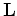, which is the linearization of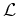with respect to velocity perturbations. This linear operator can be derived by perturbing equation 3 as follows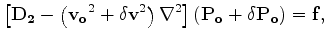(4)

where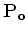and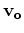are the background wavefield and velocity, respectively, and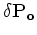is the scattered wavefield.

Equation 4 can be rewritten as the following two equations: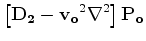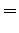(5)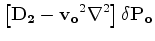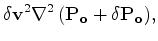(6)

which represents a nonlinear relationship between velocity perturbations and scattered wavefield. To linearize this relationship we drop the term multiplying the perturbations with each other; that is, we drop the scattered wavefield from the right hand side of equation 6 and obtain the following coupled equations:(7)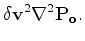(8)

The linear operatorused to compute the gradient of the FWI objective function 2 is evaluated by recursively propagating the background wavefieldand the scattered wavefieldby solving equations 7-8.

The scattered wavefieldis a linear function of the velocity perturbationsbecause equation 8 takes into account only fist order scattering. Notice that the linear operatoris itself a non linear function of the background velocity, both directly by determining the propagation speed of the scattered wavefield (left hand side in equation 8), and indirectly through the background wavefield (right hand side in equation 8).Tomographic full waveform inversion and linear modeling of multiple scatteringNext: Problems with FWI Up: Conventional Full Waveform Inversion Previous: Conventional Full Waveform Inversion

2012-10-29